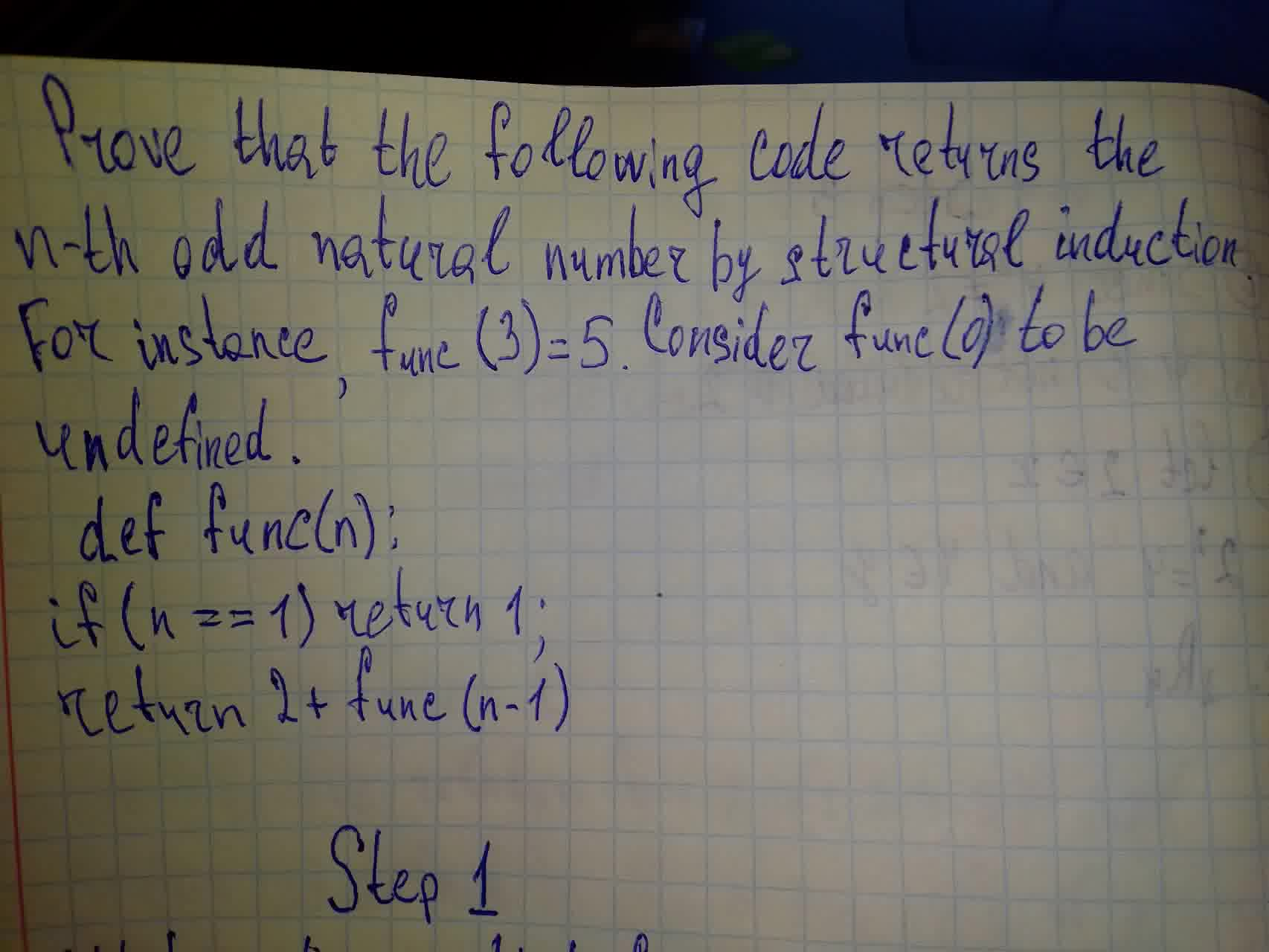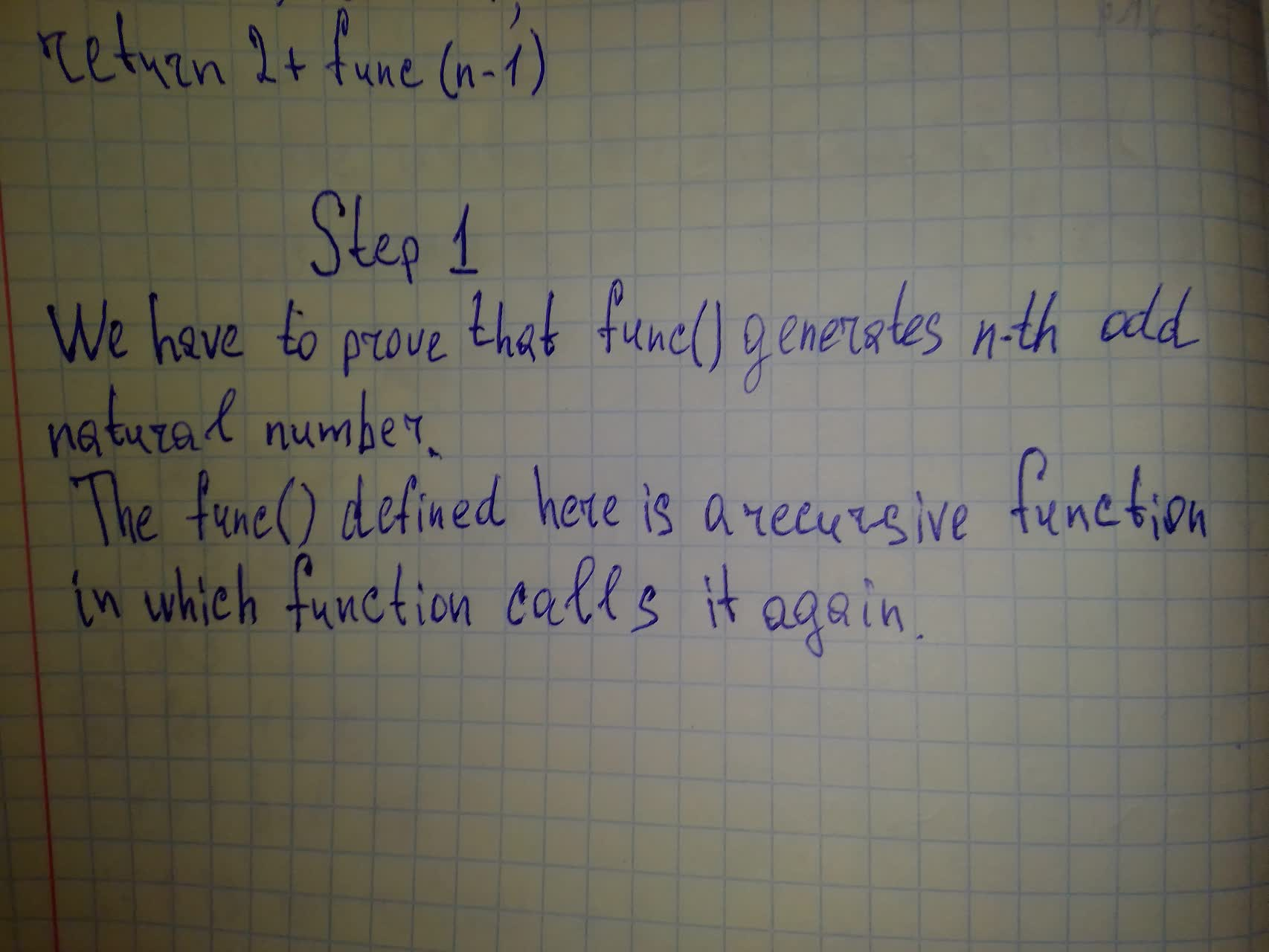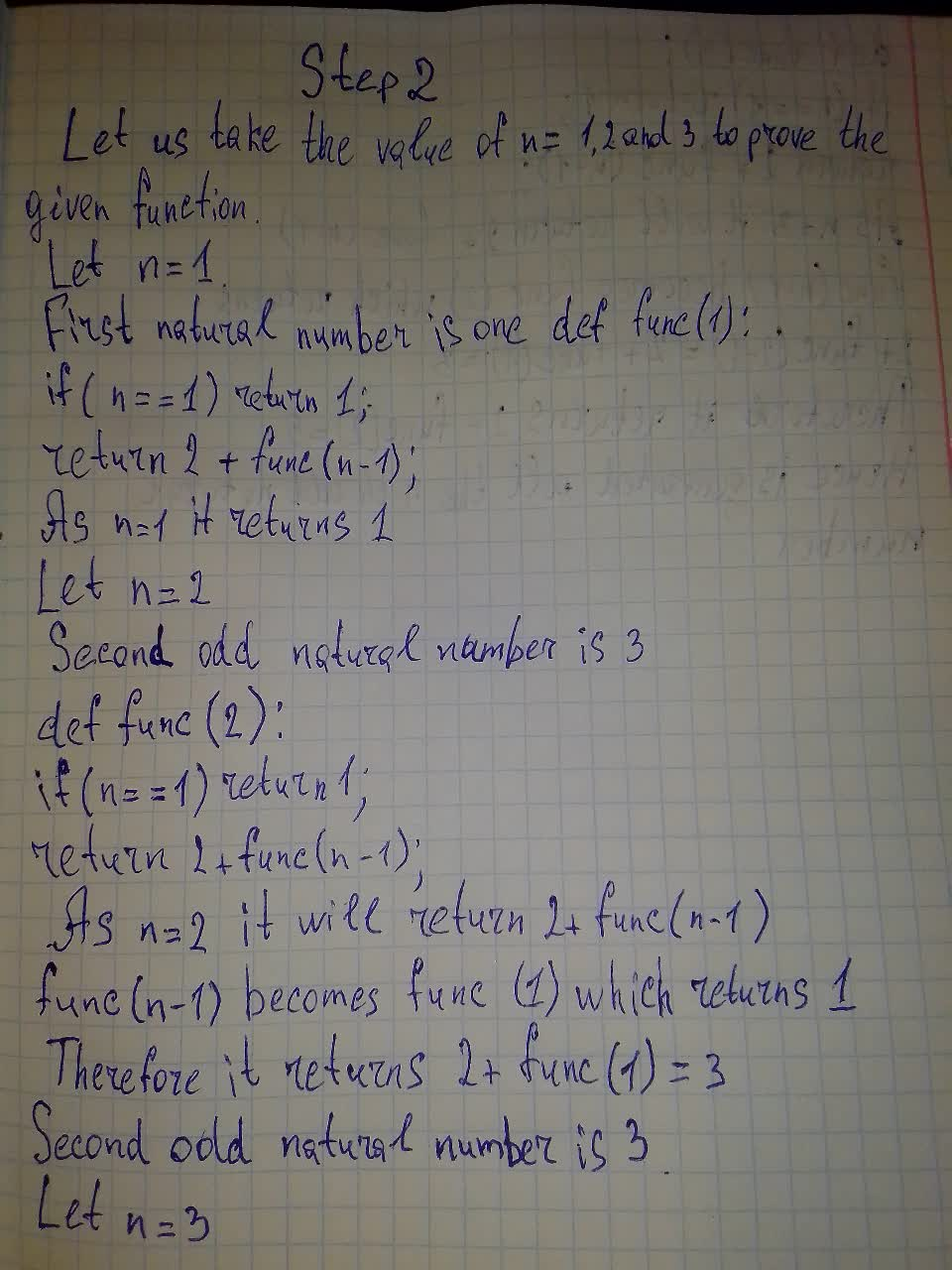Prove that the following code returns the n^{th} odd natural number by structural induction.For instance , func(3)=5.Consider func(0) to be undefinedarenceabigns 2021-07-19 Answered

b)Prove that the following code returns the $$n^{th}$$ odd natural number by structural induction.For instance , func(3)=5.Consider func(0) to be undefined.Want to know more about Discrete math?

• Questions are typically answered in as fast as 30 minutes

Solve your problem for the price of one coffee

• Math expert for every subject
• Pay only if we can solve itescumantsu

At the given condition: